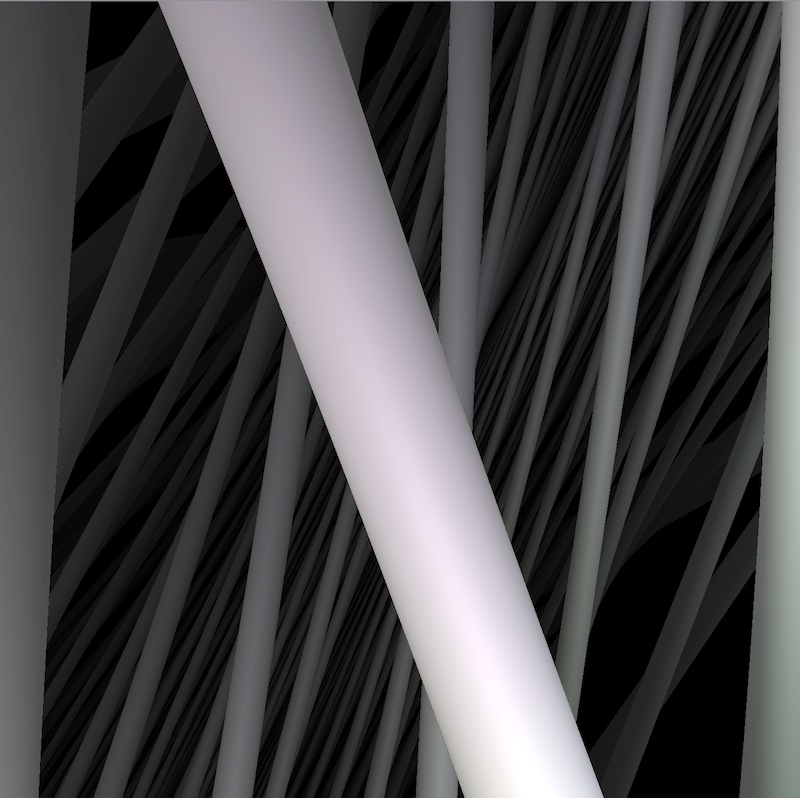# The Nil geometry

## What is Nil?

Nil is a 3-dimensional nilpotent Lie group. It can also be seen as the universal cover of the suspension of a 2-torus by a Dehn twist.

Click on the button below to reveal a concrete model of Nil.

Nil is also the 3-dimensional Heisenberg group, that is the set of matrices of the form $$\begin{bmatrix} 1 & x & z \\ 0 & 1 & y \\ 0 & 0 & 1 \end{bmatrix}$$ However this model does not highlight the symmetries of Nil. Therefore, we use a different (but isomorphic) model. As a set of points, Nil is the usual 3-dimensional space $X = \mathbb R^3$ with coordinates $(x,y,z)$. The riemanian metric on Nil is given by $$ds^2 = dx^2 + dy^2 + \left(dz - \frac 12(xdy - ydx)\right)^2.$$

Consider to points $p_1 = (x_1, y_1, z_1)$ and $p_2 = (x_2, y_2, z_2)$ in $X$. The group law in this model becomes $$p_1 \ast p_2 = \left(x_1 + x_2, y_1 + y_2, z_1 + z_2 + \frac 12 (x_1 y_2 - x_2 y_1)\right)$$ The left action of Nil on itself is an action by isometries. Its full isometry group is ${\rm Isom}(X) = {\rm Nil} \rtimes O(2)$. In particular the stabilizer of the origin $[0, 0, 0]$ contains a subgroup isomorphic to $S^1$ which corresponds to the rotations around the $z$-axis.

## Some views of Nil

Warning: Some of the real-time simulations below requires a powerful graphic card. If your computer is not fast enough, you can reduce the size of your browser window. Click on the button below to reveal the fly commands.

The default controls to fly in the scene are the following. You can choose your keyboard in the Option controls pannel in the top right corner of the window

Command QWERTY keyboard AZERTY keyboard
Yaw left a q
Yaw right d d
Pitch up w z
Pitch down s s
Roll left q a
Roll right e e
Move forward arrow up arrow up
Move backward arrow down arrow down
Move to the left arrow left arrow left
Move the the right arrow right arrow right
Move upwards ' ù
Move downwards / =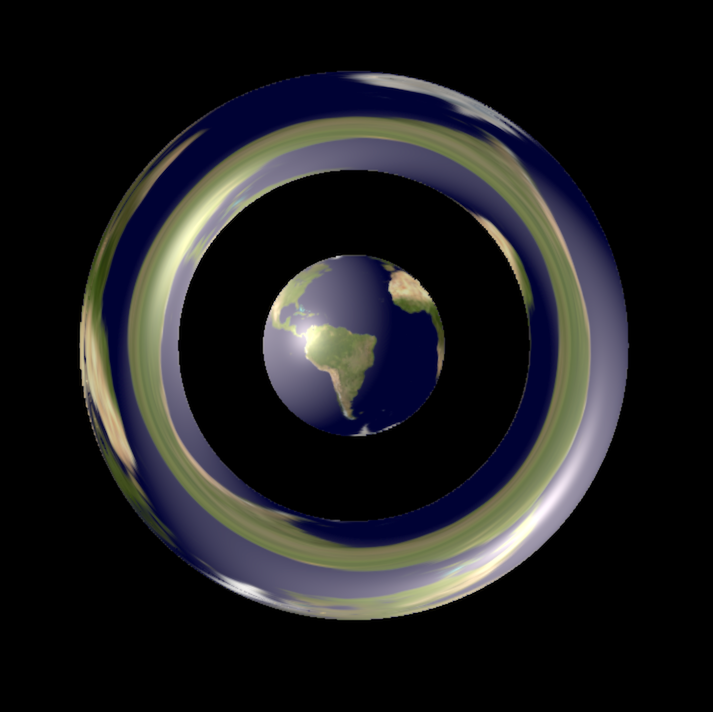##### Earth in Nil

Earth/Moon system bursting into rings?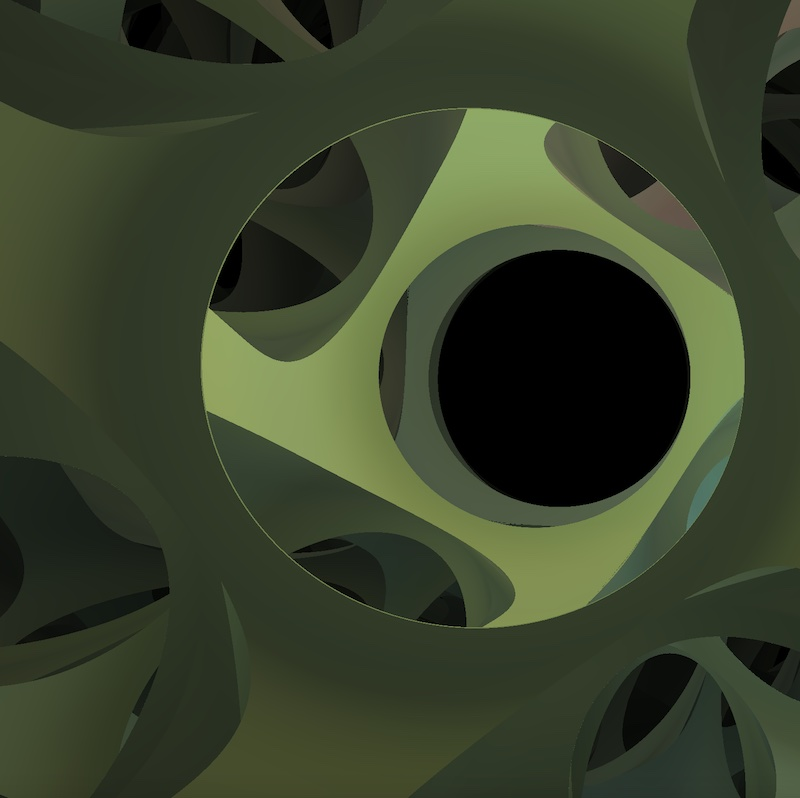##### Tiling

The effect of spiraling geodesics on vision.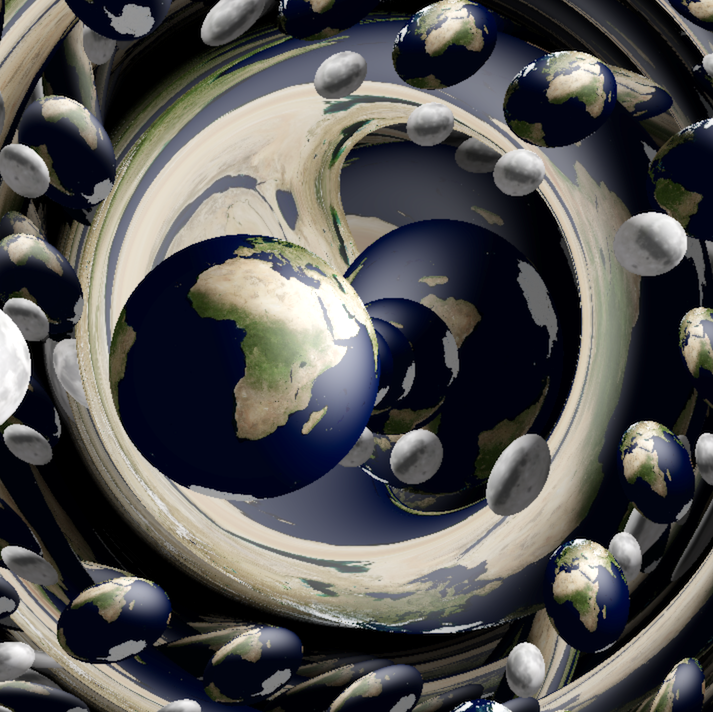##### Lattice of Spheres

The effect of spiraling geodesics on vision.

HD pictures of Nil can be found in the gallery

## Features of Nil

Some features of Nil are described in the following Bridges paper.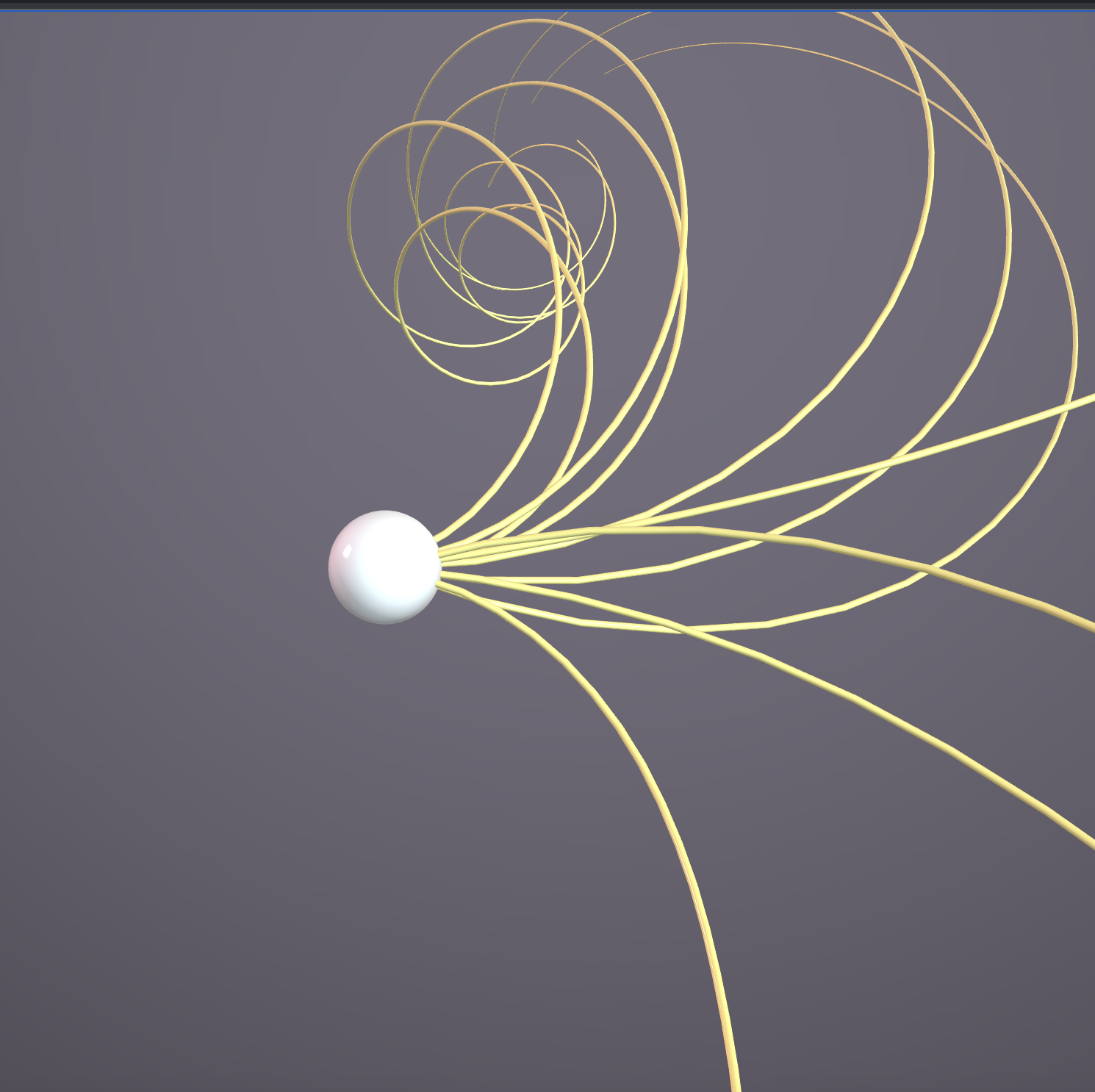##### Geodesics in Nil: Extrinsic

Spray of geodesics in various directions in rotation invariant model.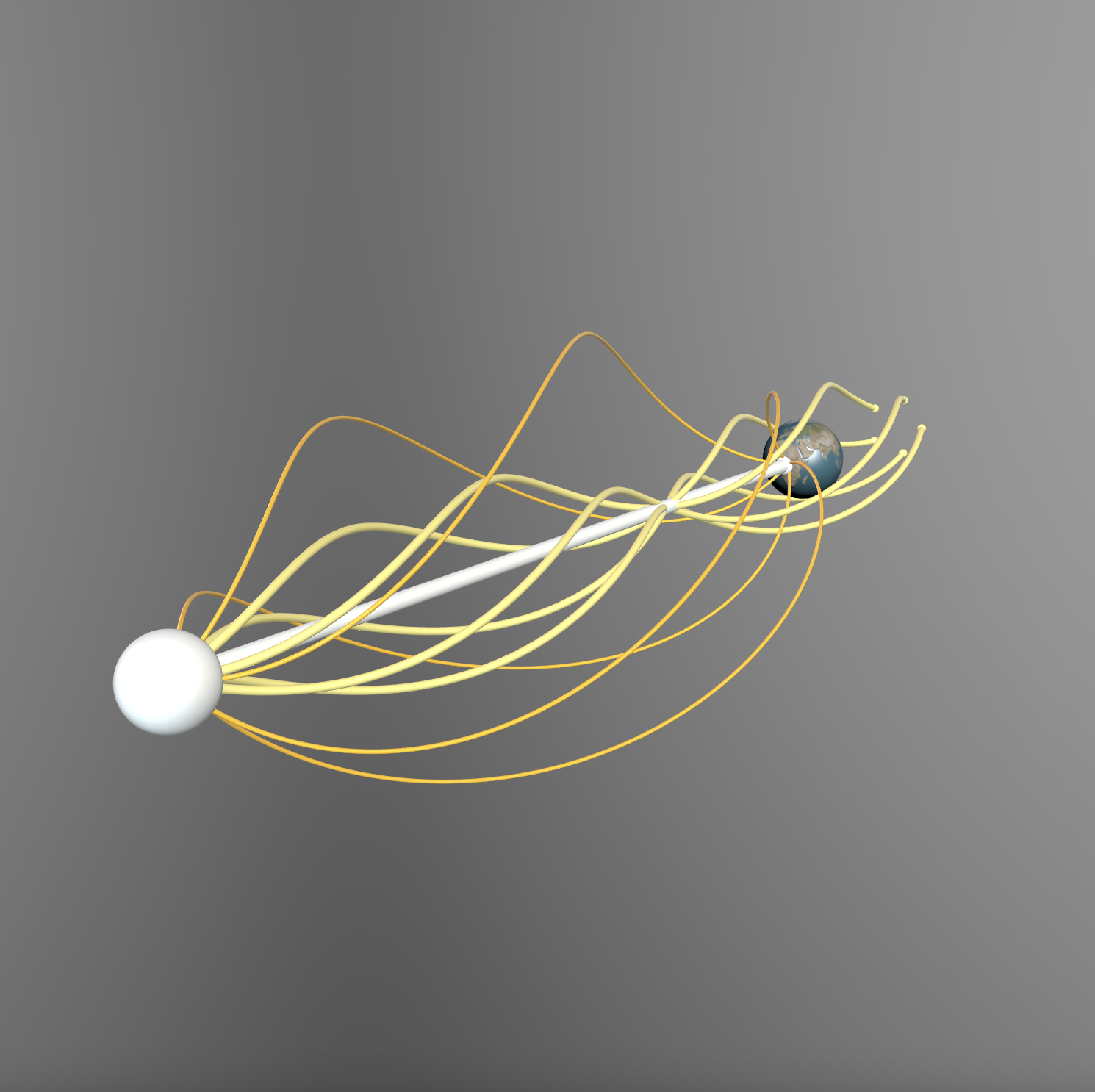##### A Sphere In Nil:Extrinsic

The effect of spiraling geodesics on vision.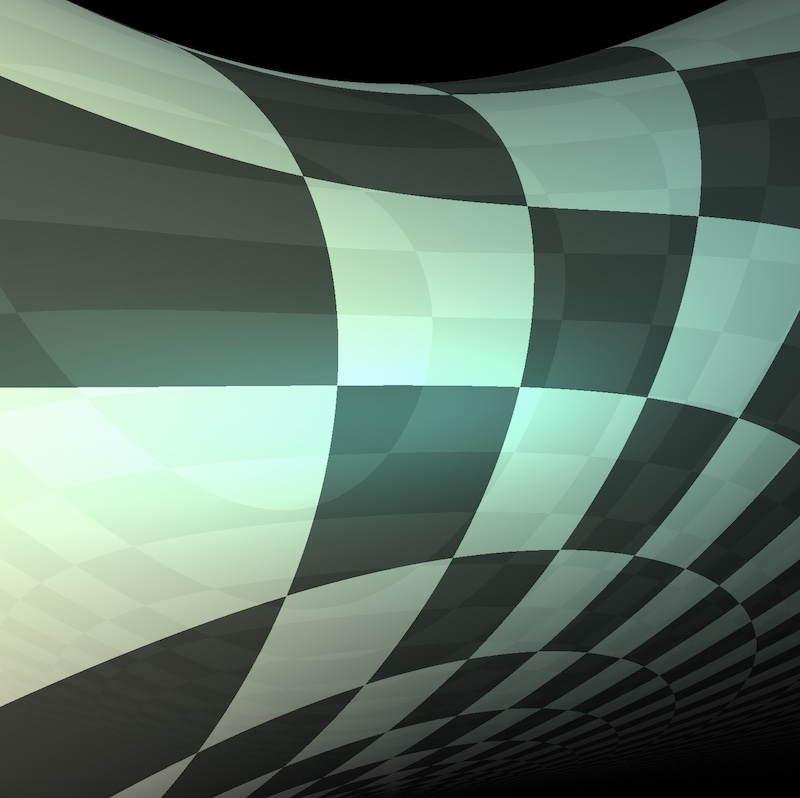##### Vertical Planes in Nil

Non-Geodesic Euclidean Planes, with an animated reflecting texture